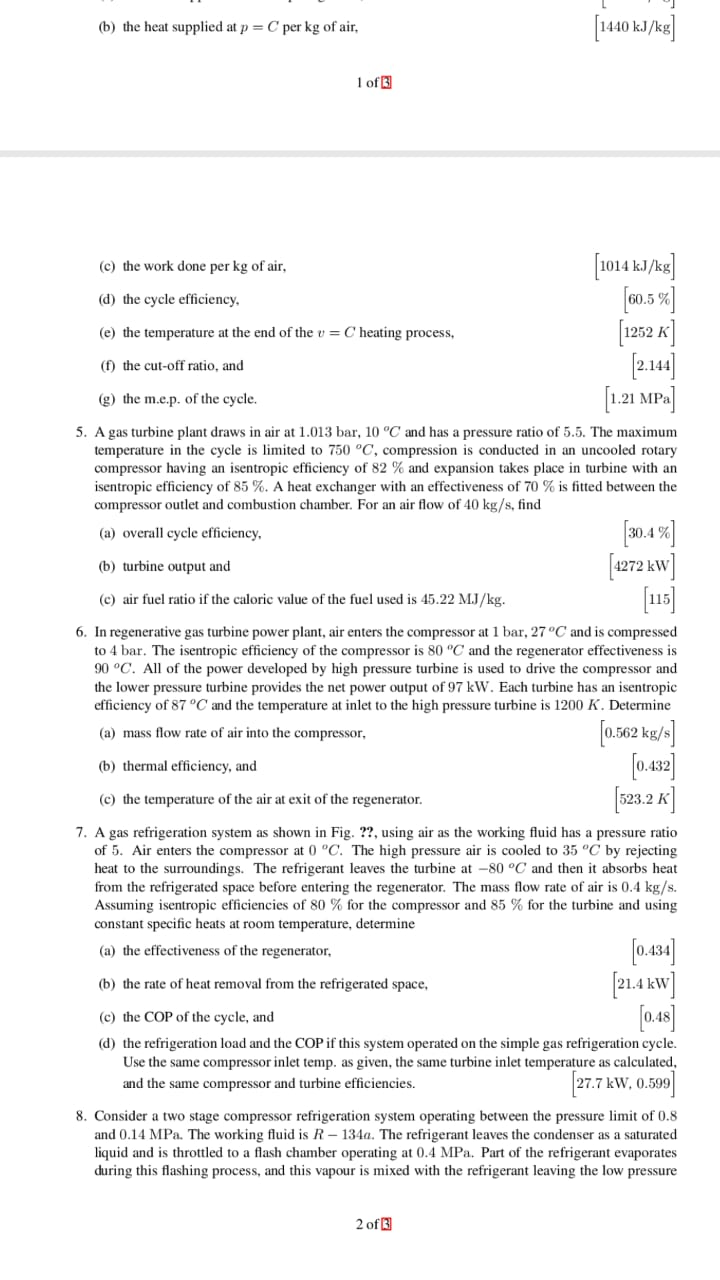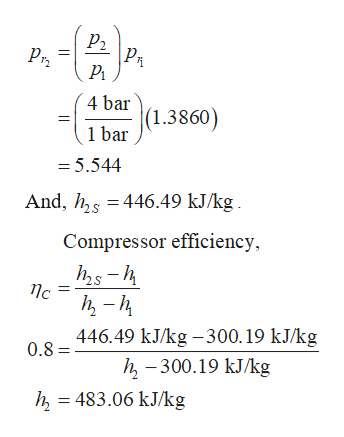# (b) the heat supplied at p C per kg of airof(c) the work done per kg of air(d) the cycle efficiency1252 K(e) the temperature at the end of theC heating process,(f) the cut-off ratio, and.21 MP-g) the m.e.p. of the cycle5. A gas turbine plant draws in air at 1.013 bar, 10 °C and has a pressure ratio of 5.5. The maximumtemperature in the cycle is limited to 750 °C, compression is conducted in an uncooled rotarycompressor having an isentropic efficiency of 82 % and expansion takes place in turbine with anisentropic efficiency of 85 %. A heat exchanger with an effectiveness of 70 % is fitted between thecompressor outlet and combustion chamber. For an air flow of 40 kg/s, find(a) overall cycle efficiency.4(b) turbine output and(c) air fuel ratio if the caloric value of the fuel used is 45.22 MJ /kg.6. In regenerative gas turbine power plant, air enters the compressor at 1 bar, 27°C and is compressedto 4 bar. The isentropic efficiency of the compressor is 80 "C and the regenerator effectiveness is90 °C. All of the power developed by high pressure turbine is used to drive the compressor andthe lower pressure turbine provides the net power output of 97 kW. Each turbine has an isentropicefficiency of 87 °C and the temperature at inlet to the high pressure turbine is 1200 K. Determine0.562 kg/s0.42]\$23.2 K(a) mass flow rate of air into the compressor(b) thermal efficiency, and(c) the temperature of the air at exit of the regenerator7. A gas refrigeration system as shown in Fig. ??, using air as the working fluid has a pressure ratioof 5. Air enters the compressor at 0 °C. The high pressure air is cooled to 35 °C by rejectingheat to the surroundings. The refrigerant leaves the turbine at -80 °C and then it absorbs heatfrom the refrigerated space before entering the regenerator. The mass flow rate of air is 0.4 kg/sAssuming isentropic efficiencies of 80% for the compressor and 85 % for the turbine and usingconstant specific heats at room temperature, determine(a) the effectiveness of the regenerator(b) the rate of heat removal from the refrigerated space,(c) the COP of the cycle, and(d) the refrigeration load and the COP if this system operated on the simple gas refrigeration cycle.Use the same compressor inlet temp, as given, the same turbine inlet temperature as calculatedand the same compressor and turbine efficiencies27.7 kW, 0.5998. Consider a two stage compressor refrigeration system operating between the pressure limit of 0.8and 0.14 MPa. The working fluid is R - 134a. The refrigerant leaves the condenser as a saturatedliquid and is throttled to a flash chamber operating at 0.4 MPa. Part of the refrigerant evaporatesduring this flashing process, and this vapour is mixed with the refrigerant leaving the low pressure2 of

Question
78 views

solve without using reduced pressure concept and also without use of referring to table of ideal gas(air) for calculating enthalpy or temperature etc.help_outlineImage Transcriptionclose(b) the heat supplied at p C per kg of air of (c) the work done per kg of air (d) the cycle efficiency 1252 K (e) the temperature at the end of the C heating process, (f) the cut-off ratio, and .21 MP- g) the m.e.p. of the cycle 5. A gas turbine plant draws in air at 1.013 bar, 10 °C and has a pressure ratio of 5.5. The maximum temperature in the cycle is limited to 750 °C, compression is conducted in an uncooled rotary compressor having an isentropic efficiency of 82 % and expansion takes place in turbine with an isentropic efficiency of 85 %. A heat exchanger with an effectiveness of 70 % is fitted between the compressor outlet and combustion chamber. For an air flow of 40 kg/s, find (a) overall cycle efficiency .4 (b) turbine output and (c) air fuel ratio if the caloric value of the fuel used is 45.22 MJ /kg. 6. In regenerative gas turbine power plant, air enters the compressor at 1 bar, 27°C and is compressed to 4 bar. The isentropic efficiency of the compressor is 80 "C and the regenerator effectiveness is 90 °C. All of the power developed by high pressure turbine is used to drive the compressor and the lower pressure turbine provides the net power output of 97 kW. Each turbine has an isentropic efficiency of 87 °C and the temperature at inlet to the high pressure turbine is 1200 K. Determine 0.562 kg/s 0.42] \$23.2 K (a) mass flow rate of air into the compressor (b) thermal efficiency, and (c) the temperature of the air at exit of the regenerator 7. A gas refrigeration system as shown in Fig. ??, using air as the working fluid has a pressure ratio of 5. Air enters the compressor at 0 °C. The high pressure air is cooled to 35 °C by rejecting heat to the surroundings. The refrigerant leaves the turbine at -80 °C and then it absorbs heat from the refrigerated space before entering the regenerator. The mass flow rate of air is 0.4 kg/s Assuming isentropic efficiencies of 80% for the compressor and 85 % for the turbine and using constant specific heats at room temperature, determine (a) the effectiveness of the regenerator (b) the rate of heat removal from the refrigerated space, (c) the COP of the cycle, and (d) the refrigeration load and the COP if this system operated on the simple gas refrigeration cycle. Use the same compressor inlet temp, as given, the same turbine inlet temperature as calculated and the same compressor and turbine efficiencies 27.7 kW, 0.599 8. Consider a two stage compressor refrigeration system operating between the pressure limit of 0.8 and 0.14 MPa. The working fluid is R - 134a. The refrigerant leaves the condenser as a saturated liquid and is throttled to a flash chamber operating at 0.4 MPa. Part of the refrigerant evaporates during this flashing process, and this vapour is mixed with the refrigerant leaving the low pressure 2 of fullscreen
check_circle

Step 1

Consider the T-S diagram for the regenerative gas turbine power plant.

Here, some assumptions are to be made like, each component is analysed as a control volume at steady state, the compressor, turbines and regenerator shows adiabatic behaviour and the working fluid is taken air but shows ideal behaviour.

Step 2

Observe the T-S diagram of the turbine power plant and consider the State 1 where the air enters the compressor. Here,

Step 3

Further, observe the next step, where the isentropic compression takes place. Determine the pressure at the Step 2S wil...help_outlineImage TranscriptioncloseР. PR Pry 4 bar (1.3860) 1 bar = 5.544 And, hs 446.49 kJ/kg Compressor efficiency nc 446.49 kJ/kg -300.19 kJ/kg h-300.19 kJ/kg 483.06 kJ/kg 0.8= fullscreen

### Want to see the full answer?

See Solution

#### Want to see this answer and more?

Solutions are written by subject experts who are available 24/7. Questions are typically answered within 1 hour.*

See Solution
*Response times may vary by subject and question.
Tagged in

### Mechanical Engineering